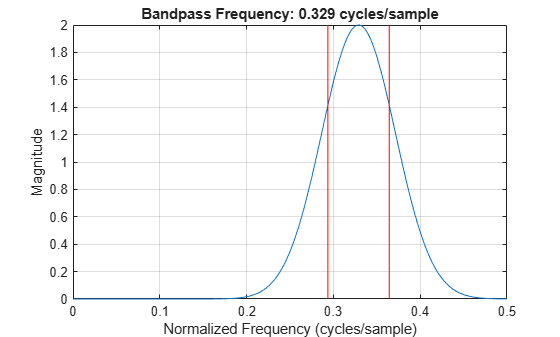# powerbw

CWT filter bank 3 dB bandwidths

## Syntax

``bw = powerbw(fb)``

## Description

example

````bw = powerbw(fb)` returns 3 dB (half-power) bandwidths for the wavelet filters in the filter bank `fb`. `bw` is a Ns-by-4 MATLAB® `table`, where Ns is the number of wavelet bandpass frequencies (equal to the number of scales). For every filter in `fb`, the table contains the corresponding bandpass frequency, the 3 dB bandwidth, and the lower frequency and upper frequency limits of the 3 dB bandwidth.The 3 dB bandwidth limits mark where the filter power is half its peak value. The magnitude frequency response at the limits is equal to 1/√2 times the peak magnitude. Since the passbands in `fb` are normalized with peak magnitudes approximately equal to 2, the magnitude frequency response at each limit is approximately equal to 2/√2. The 3 dB bandwidth is also known as the half-power bandwidth because $20{\mathrm{log}}_{10}\frac{1}{\sqrt{2}}\approx -3.$```

## Examples

collapse all

Create a CWT filter bank.

`fb = cwtfilterbank;`

Obtain the 3 dB (half-power) bandwidths of the filter bank. Obtain the frequency responses of the wavelets.

```bw = powerbw(fb); [psidft,f] = freqz(fb);```

Choose a wavelet bandpass filter from the filter bank. Extract from the table `bw` the 3 dB limits of the bandpass filter.

```wv = 5; frq = bw.Frequencies(wv); lfb = bw.LowFrequencyBorder(wv); hfb = bw.HighFrequencyBorder(wv);```

Plot the frequency response and 3 dB limits. Since the frequency response is scaled to have a maximum value equal to 2, inspect the plot to confirm the lower and upper frequency borders intersect the frequency response at $\sqrt{2}$.

```plot(f,psidft(wv,:)) grid on hold on plot([lfb lfb],[0 2],'r') plot([hfb hfb],[0 2],'r') xlabel('Normalized Frequency (cycles/sample)') ylabel('Magnitude') title(['Bandpass Frequency: ' num2str(frq) ' cycles/sample'])```## Input Arguments

collapse all

Continuous wavelet transform (CWT) filter bank, specified as a `cwtfilterbank` object.

## Output Arguments

collapse all

3 dB (half-power) bandwidths, returned as an Ns-by-4 `table`, where Ns is the number of wavelet bandpass frequencies (equal to the number of scales). The table has four variables:

Bandpass frequency, returned as a positive scalar (see `centerFrequencies`).

Data Types: `double`

Half-power bandwidth, returned as a positive scalar.

Data Types: `double`

Lower frequency edge of the 3 dB bandwidth, returned as a positive scalar.

Data Types: `double`

High frequency edge of the 3 dB bandwidth, returned as a positive scalar.

Data Types: `double`

Data Types: `table`